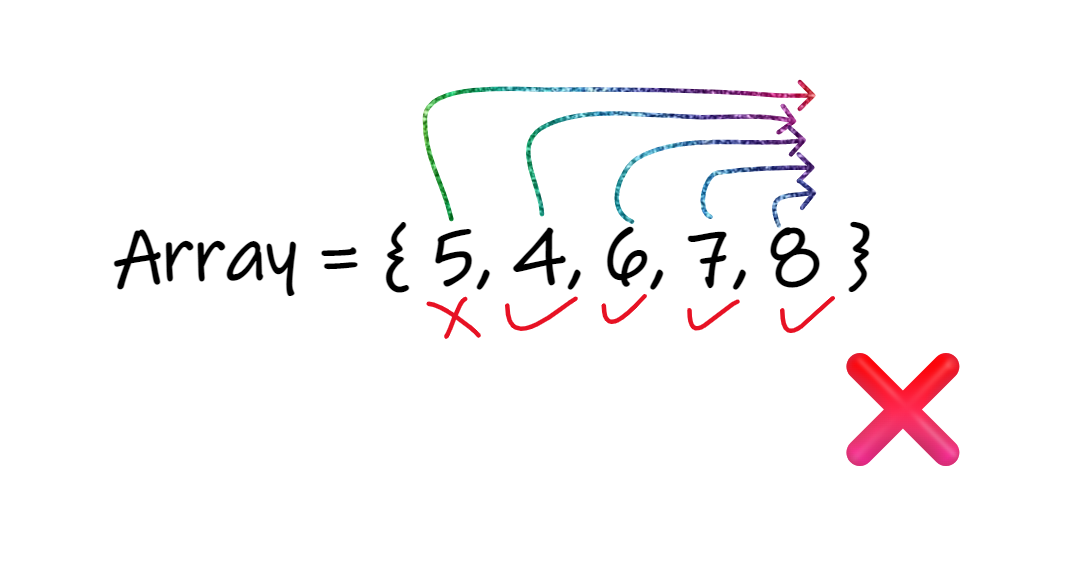# Check if an Array is Sorted

Problem Statement: Given an array of size n, write a program to check if the given array is sorted in (ascending / Increasing / Non-decreasing) order or not. If the array is sorted then return True, Else return False.

Note: Two consecutive equal values are considered to be sorted.

Examples
```Example 1:
Input: N = 5, array[] = {1,2,3,4,5}
Output: True.
Explanation: The given array is sorted i.e Every element in the array is smaller than or equals to its next values, So the answer is True.Example 2:
Input: N = 5, array[] = {5,4,6,7,8}
Output: False.
Explanation: The given array is Not sorted i.e Every element in the array is not smaller than or equal to its next values, So the answer is False.

Here element 5 is not smaller than or equal to its future elements.```

Disclaimer: Don’t jump directly to the solution, try it out yourself first.

Brute Force Approach Optimal Approach
Brute Force Approach
Algorithm / Intuition

### Approach: Brute Force

• The idea is pretty simple here, We will start with the element at the 0th index, and will compare it with all of its future elements that are present in the array.
• If the picked element is smaller than or equal to all of its future values then we will move to the next Index/element until the whole array is traversed.
• If any of the picked elements is greater than its future elements, Then simply we will return False.
• If the size of the array is Zero or One i.e ( N = 0 or N = 1 ) or the entire array is traversed successfully then we will simply return True.
Code
``````
#include <bits/stdc++.h>

using namespace std;

bool isSorted(int arr[], int n) {
for (int i = 0; i < n; i++) {
for (int j = i + 1; j < n; j++) {
if (arr[j] < arr[i])
return false;
}
}

return true;
}

int main() {

int arr[] = {1, 2, 3, 4, 5}, n = 5;
bool ans = isSorted(arr, n);
if (ans) cout << "True" << endl;
else cout << "False" << endl;
return 0;
}
```
```

Output: True

``````
class TUF {
static boolean isSorted(int arr[], int n) {
for (int i = 0; i < n; i++) {
for (int j = i + 1; j < n; j++) {
if (arr[j] < arr[i])
return false;
}
}

return true;
}

public static void main(String args[]) {
int arr[] = {1, 2, 3, 4, 5}, n = 5;

System.out.println(isSorted(arr, n));
}
}
```
```

Output: True

``````
def isSorted(arr, n):
for i in range(n):
for j in range(i + 1, n):
if arr[j] < arr[i]:
return False

return True

if __name__ == "__main__":
arr = [1, 2, 3, 4, 5]
n = len(arr)
ans = isSorted(arr, n)
if ans:
print("True")
else:
print("False")
```
```

Output: True

``````
function isSorted(arr) {
for (let i = 0; i < arr.length; i++) {
for (let j = i + 1; j < arr.length; j++) {
if (arr[j] < arr[i])
return false;
}
}

return true;
}

const arr = [1, 2, 3, 4, 5];
const ans = isSorted(arr);
if (ans) console.log("True");
else console.log("False");

```
```

Output: True

Complexity Analysis

Time Complexity: O(N^2)

Space Complexity: O(1)

Optimal Approach
Algorithm / Intuition

### Approach: Efficient (Single traversal)

• As we know that for a sorted array the previous of every element is smaller than or equal to its current element.
• So, Through this, we can conclude that if the previous element is smaller than or equal to the current element then. Then we can say that the two elements are sorted. If the condition is true for the entire array then the array is sorted.
• We will check every element with its previous element if the previous element is smaller than or equal to the current element then we will move to the next index.
• If the whole array is traversed successfully or the size of the given array is zero or one (i.e N = 0 or N = 1). Then we will return True else return False.
Code
``````
#include<bits/stdc++.h>

using namespace std;

bool isSorted(int arr[], int n) {
for (int i = 1; i < n; i++) {
if (arr[i] < arr[i - 1])
return false;
}

return true;
}

int main() {

int arr[] = {1, 2, 3, 4, 5}, n = 5;

printf("%s", isSorted(arr, n) ? "True" : "False");

}
```
```

Output: True

``````
class TUF {
static boolean isSorted(int arr[], int n) {
for (int i = 1; i < n; i++) {
if (arr[i] < arr[i - 1])
return false;
}

return true;
}

public static void main(String args[]) {
int arr[] = {1, 2, 3, 4, 5}, n = 5;

System.out.println(isSorted(arr, n));
}
}
```
```

Output: True

``````
def isSorted(arr, n):
for i in range(1, n):
if arr[i] < arr[i - 1]:
return False
return True

if __name__ == "__main__":
arr = [1, 2, 3, 4, 5]
n = 5
print("True" if isSorted(arr, n) else "False")
```
```

Output: True

``````
function isSorted(arr) {
for (let i = 1; i < arr.length; i++) {
if (arr[i] < arr[i - 1])
return false;
}

return true;
}

const arr = [1, 2, 3, 4, 5];

console.log(isSorted(arr) ? "True" : "False");
```
```

Output: True

Complexity Analysis

Time Complexity: O(N)

Space Complexity: O(1)

Video Explanation